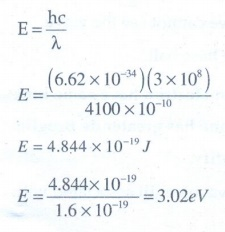Home | | Physics 12th Std | Multiple Choice Questions

# Multiple Choice Questions

Physics : Dual Nature of Radiation and Matter: Book Back Choose the correct Answers with Solution, Multiple choice questions with Answers and Solution

I Multiple Choice Questions

1. The wavelength ╬╗e of an electron and ╬╗p of a photon of same energy E are related by

a. ╬╗p ŌłØ ╬╗e

b. ╬╗p ŌłØ ŌłÜ╬╗e

c. ╬╗p ŌłØ 1/ŌłÜ╬╗e

d. ╬╗p ŌłØ ╬╗2e

Solution:

de Broglie wavelength for an electron

╬╗e= h / ŌłÜ(2mE) ;

╬╗e ŌłØ 1/ŌłÜE ;

╬╗e2 ŌłØ 1/E  ------------- (1)

For photon

╬╗p= hc / E ;

╬╗p ŌłØ 1/E  ------------- (2)

From equation (1) and (2), we have So,

╬╗p ŌłØ ╬╗e2

2. In an electron microscope, the electrons are accelerated by a voltage of 14 kV. If the voltage is changed to 224 kV, then the de Broglie wavelength associated with the electrons would

a. increase by 2 times

b. decrease by 2 times

c. decrease by 4 times

d. increase by 4 times

Solution:

deBroglie wavelength ╬╗ ŌłØ 1/ŌłÜV

╬╗1/╬╗2 = ŌłÜ(V2/V1) = ŌłÜ(224/14) = ŌłÜ16 = 4

╬╗1 = 4╬╗2

╬╗2 = ╬╗1/4

3. A particle of mass 3 ├Ś 10ŌĆō6 g has the same wavelength as an electron moving with a velocity 6├Ś106 m sŌłÆ1 . The velocity of the particle is

a. 1.82├Ś10ŌłÆ18 m sŌłÆ1

b. 9├Ś10ŌłÆ2 m sŌłÆ1

c. 3├Ś10ŌłÆ31m sŌłÆ1

d. 1.82├Ś10ŌłÆ15 m sŌłÆ1

Solution:4. When a metallic surface is illuminated with radiation of wavelength ╬╗, the stopping potential is V. If the same surface is illuminated with radiation of wavelength 2╬╗, the stopping potential is V/4. The threshold wavelength for the metallic surface is

a. 4╬╗

b. 5╬╗

c. 5/2  ╬╗

d. 3╬╗

Solution:5. If a light of wavelength 330 nm is incident on a metal with work function 3.55 eV, the electrons are emitted. Then the wavelength of the emitted electron is (Take h = 6.6 ├Ś 10ŌĆō34 Js)

a. < 2.75├Ś10ŌłÆ9 m

b. Ōēź 2.75├Ś10ŌłÆ9 m

c. Ōēż 2.75├Ś10ŌłÆ12 m

d. < 2.5├Ś10ŌłÆ10 m

Solution:6. A photoelectric surface is illuminated successively by monochromatic light of wavelength ╬╗ and ╬╗/2. If the maximum kinetic energy of the emitted photoelectrons in the second case is 3 times that in the first case, the work function at the surface of material is

a) hc/╬╗

b) 2hc/ ╬╗

c) hc/3╬╗

d) hc/2╬╗

Solution:7. In photoelectric emission, a radiation whose frequency is 4 times threshold frequency of a certain metal is incident on the metal. Then the maximum possible velocity of the emitted electron will beSolution:

From Enistein's equation8. Two radiations with photon energies 0.9 eV and 3.3 eV respectively are falling on a metallic surface successively. If the work function of the metal is 0.6 eV, then the ratio of maximum speeds of emitted electrons will be

a) 1:4

b) 1:3

c) 1:1

d) 1:9

Solution:9. A light source of wavelength 520 nm emits 1.04 ├Ś 1015 photons per second while the second source of 460 nm  produces 1.38 ├Ś 1015 photons per second. Then the ratio of power of second source  to that of first source is

a) 1.00

b) 1.02

c) 1.5

d) 0.98

Solution:10. The mean wavelength of light from sun is taken to be 550 nm and its mean power is 3.8 ├Ś 1026W. The number of photons received by the human eye per second on the average from sunlight is of the order of

a) 1045

b) 1042

c) 1054

d) 1051

Solution:11. The threshold wavelength for a metal surface whose photoelectric work function is 3.313 eV is

a) 4125├ģ

b) 3750 ├ģ

c) 6000 ├ģ

d) 2062.5├ģ

Solution:12. A light of wavelength 500 nm is incident on a sensitive plate of photoelectric work function 1.235 eV. The kinetic energy of the photo electrons emitted is be (Take h = 6.6 ├Ś 10ŌĆō34 Js)

a) 0.58 eV

b) 2.48 eV

c) 1.24 eV

d) 1.16 eV

Solution:13. Photons of wavelength ╬╗ are incident on a metal. The most energetic electrons ejected from the metal are bent into a circular arc of radius R by a perpendicular magnetic field having magnitude B. The work function of the metal isSolution:

Centripetal force (Fc.p) = Magnetic force (FB)14. The work functions for metals A, B and C are 1.92 eV, 2.0 eV and 5.0 eV respectively. The metals which will emit photoelectrons for a radiation of wavelength 4100 ├ģ is/are

a. A only

b. both A and B

c. all these metals

d. none

Solution:

The energy of the light with the wavelength 4100 ├Ś 10-10 mBoth (A) and (B) emits the electrons, because its work function is less than 3.02eV.

15. Emission of electrons by the absorption of heat energy is called _______ emission.

a. photoelectric

b. field

c. thermionic

d. secondary